Courses

# Problems for practice Mathematics Notes | EduRev

## Mathematics : Problems for practice Mathematics Notes | EduRev

The document Problems for practice Mathematics Notes | EduRev is a part of the Mathematics Course Algebra for IIT JAM Mathematics.
All you need of Mathematics at this link: Mathematics

For problems 1 & 2 list the first 5 terms of the sequence.
1.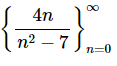Solution. There really isn’t all that much to this problem. All we need to do is, starting at n=0, plug in the first five values of n into the formula for the sequence terms. Doing that gives,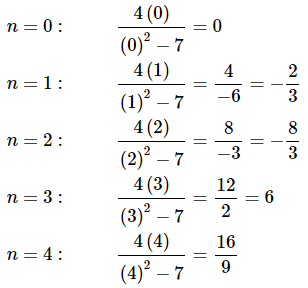So, the first five terms of the sequence are,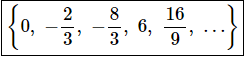Note that we put the formal answer inside the braces to make sure that we don’t forget that we are dealing with a sequence and we made sure and included the “…” at the end to reminder ourselves that there are more terms to this sequence that just the five that we listed out here.
2.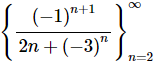Solution. There really isn’t all that much to this problem. All we need to do is, starting at n=2, plug in the first five values of n into the formula for the sequence terms. Doing that gives,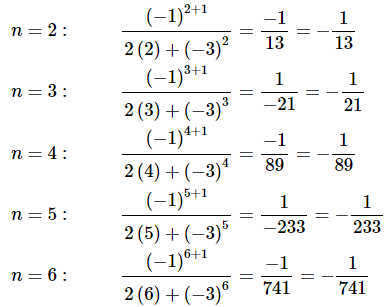So, the first five terms of the sequence are,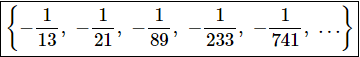Note that we put the formal answer inside the braces to make sure that we don’t forget that we are dealing with a sequence and we made sure and included the “…” at the end to reminder ourselves that there are more terms to this sequence that just the five that we listed out here.
For problems 3 – 6 determine if the given sequence converges or diverges. If it converges what is its limit?
3.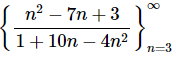Solution. To answer this all we need is the following limit of the sequence terms.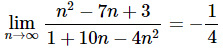You do recall how to take limits at infinity right? If not you should go back into the Calculus I material do some refreshing on limits at infinity as well at L’Hospital’s rule.
We can see that the limit of the terms existed and was a finite number and so we know that the sequence converges and its limit is −1/4.
4.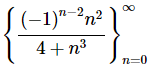Solution. To answer this all we need is the following limit of the sequence terms.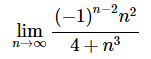However, because of the (−1)n−2 we can’t compute this limit using our knowledge of computing limits from Calculus I.
Recall however, that we had a nice Fact in the notes from this section that had us computing not the limit above but instead computing the limit of the absolute value of the sequence terms.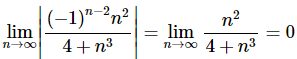This is a limit that we can compute because the absolute value got rid of the alternating sign, i.e. the (−1)n+2.
Now, by the Fact from class we know that because the limit of the absolute value of the sequence terms was zero (and recall that to use that fact the limit MUST be zero!) we also know the following limit.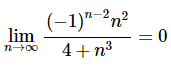We can see that the limit of the terms existed and was a finite number and so we know that the sequence converges and its limit is zero.
5.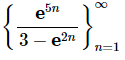Solution. To answer this all we need is the following limit of the sequence terms.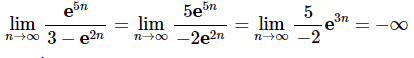You do recall how to use L’Hospital’s rule to compute limits at infinity right? If not you should go back into the Calculus I material do some refreshing.
We can see that the limit of the terms existed and but was infinite and so we know that the sequence diverges.
6.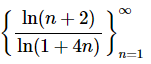Solution. To answer this all we need is the following limit of the sequence terms.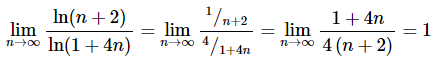You do recall how to use L’Hospital’s rule to compute limits at infinity right? If not, you should go back into the Calculus I material do some refreshing.
We can see that the limit of the terms existed and was a finite number and so we know that the sequence converges and its limit is one.

For each of the following problems determine if the sequence is increasing, decreasing, not monotonic, bounded below, bounded above and/or bounded.
1.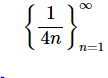Solution. For this problem let’s get the bounded information first as that seems to be pretty simple.
First note that because both the numerator and denominator are positive then the quotient is also positive and so we can see that the sequence must be bounded below by zero.
Next let’s note that because we are starting with n=1 the denominator will always be 4n≥4>1 and so we can also see that the sequence must be bounded above by one. Note that, in this case, this not the “best” upper bound for the sequence but the problem didn’t ask for that. For this sequence we’ll be able to get a better one once we have the increasing/decreasing information.
Because the sequence is bounded above and bounded below the sequence is also bounded.
Step 2 For the increasing/decreasing information we can see that, for our range of n≥1, we have,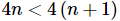and so,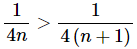If we define an=1/4n this in turn tells us that an>an+1 for all n≥1 and so the sequence is decreasing and hence monotonic.
Note that because we have now determined that the sequence is decreasing we can see that the “best” upper bound would be the first term of the sequence or, 1/4.
2.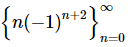Solution. For this problem let’s get the increasing/decreasing information first as that seems to be pretty simple and will help at least a little bit with the bounded information.
In this case let’s just write out the first few terms of the sequence.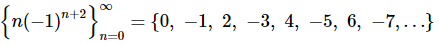Just from the first three terms we can see that this sequence is not an increasing sequence and it is not a decreasing sequence and therefore is not monotonic.
Step 2 Now let’s see what bounded information we can get.
From the first few terms of the sequence we listed out above we can see that each successive term will get larger and change signs. Therefore, there cannot be an upper or a lower bound for the sequence. No matter what value we would try to use for an upper or a lower bound all we would need to do is take n large enough and we would eventually get a sequence term that would go past the proposed bound.
Therefore, this sequence is not bounded.
3.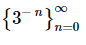Solution. For this problem let’s get the increasing/decreasing information first as that seems to be pretty simple and will help at least a little bit with the bounded information.
We’ all agree that, for our range of n≥0, we have,
n<n+1
This in turn gives,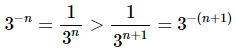So, if we define an=3−n we have an>an+1 for all n≥0 and so the sequence is decreasing and hence is also monotonic.
Step 2 Now let’s see what bounded information we can get.
First, it is hopefully obvious that all the terms are positive and so the sequence is bounded below by zero.
Next, we saw in the first step that the sequence was decreasing and so the first term will be the largest term and so the sequence is bounded above by 3−(0)=1 (i.e. the n=0 sequence term).
Therefore, because this sequence is bounded below and bounded above the sequence is bounded.
4.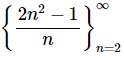Solution. For this problem let’s get the increasing/decreasing information first.
For Problems 1 – 3 in this section it was easy enough to just ask what happens if we increase n to determine the increasing/decreasing information for this problem. However, in this case, increasing n will increase both the numerator and denominator and so it would be somewhat more difficult to do that analysis here.
Therefore, we will resort to some quick Calculus I to determine increasing/decreasing information. We can define the following function and take its derivative.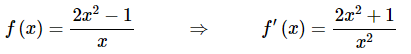We can clearly see that the derivative will always be positive for x≠0 and so the function is increasing for x≠0. Therefore, because the function values are the same as the sequence values when x is an integer we can see that the sequence, which starts at n=2, must also be increasing and hence it is also monotonic.
Step 2 Now let’s see what bounded information we can get.
First, it is hopefully obvious that all the terms are positive for our range of n≥2 and so the sequence is bounded below by zero. We could also use the fact that the sequence is increasing the first term would have to be the smallest term in the sequence and so a better lower bound would be the first sequence term which is 7/2. Either would work for this problem.
Now let’s see what we can determine about an upper bound (provided it has one of course…).
We know that the function is increasing but that doesn’t mean there is no upper bound. Take a look at Problems 1 and 3 above. Each of those were decreasing sequences and yet they had a lower bound. Do not make the mistake of assuming that an increasing sequence will not have an upper bound or a decreasing sequence will not have a lower bound. Sometimes they will and sometimes they won’t.
For this sequence we’ll need to approach any potential upper bound a little differently than the previous problems. Let’s first compute the following limit of the terms,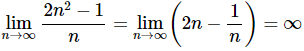Since the limit of the terms is infinity we can see that the terms will increase without bound. Therefore, in this case, there really is no upper bound for this sequence. Please remember the warning above however! Just because this increasing sequence had no upper bound does not mean that every increasing sequence will not have an upper bound.
Finally, because this sequence is bounded below but not bounded above the sequence is not bounded.
5.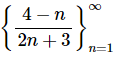Solution. For this problem let’s get the increasing/decreasing information first.
For Problems 1 – 3 in this section it was easy enough to just ask what happens if we increase n to determine the increasing/decreasing information for this problem. However, in this case, increasing n will increase both the numerator and denominator and so it would be somewhat more difficult to do that analysis here.
Therefore, we will resort to some quick Calculus I to determine increasing/decreasing information. We can define the following function and take its derivative.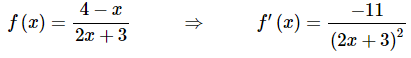We can clearly see that the derivative will always be negative for x≠−3/2 and so the function is decreasing for x≠−3/2. Therefore, because the function values are the same as the sequence values when x is an integer we can see that the sequence, which starts at n=1, must also be decreasing and hence it is also monotonic.
Step 2 Now let’s see what bounded information we can get.
First, because the sequence is decreasing we can see that the first term of the sequence will be the largest and hence will also be an upper bound for the sequence. So, the sequence is bounded above by 3/5 (i.e. the n=1 sequence term).
Next let’s look for the lower bound (if it exists). For this problem let’s first take a quick look at the limit of the sequence terms. In this case the limit of the sequence terms is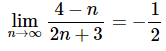Recall what this limit tells us about the behavior of our sequence terms. The limit means that as n→∞ the sequence terms must be getting closer and closer to −1/2.
Now, for a second, let’s suppose that that −1/2 is not a lower bound for the sequence terms and let’s also keep in mind that we’ve already determined that the sequence is decreasing (means that each successive term must be smaller than (i.e. below) the previous one…).
So, if −1/2 is not a lower bound then we know that somewhere there must be sequence terms below (or smaller than) −1/2. However, because we also know that terms must be getting closer and closer to −1/2 and we’ve now assumed there are terms below −1/2 the only way for that to happen at this point is for at least a few sequence terms to increase up towards −1/2(remember we’ve assumed there are terms below this!). That can’t happen however because we know the sequence is a decreasing sequence.
Okay, what was the point of all this? Well recall that we got to this apparent contradiction to the decreasing nature of the sequence by first assuming that −1/2 was not a lower bound. Since making this assumption led us to something that can’t possibly be true that in turn means that −1/2 must in fact be a lower bound since we’ve shown that sequence terms simply cannot go below this value!
Therefore, the sequence is bounded below by −1/2.
Finally, because this sequence is both bounded above and bounded below the sequence is bounded.
Before leaving this problem a quick word of caution. The limit of a sequence is not guaranteed to be a bound (upper or lower) for a sequence. It will only be a bound under certain circumstances and so we can’t simply compute the limit and assume it will be a bound for every sequence! Can you see a condition that will allow the limit to be a bound?
6.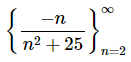Solution. For this problem let’s get the increasing/decreasing information first.
For Problems 1 – 3 in this section it was easy enough to just ask what happens if we increase n to determine the increasing/decreasing information for this problem. However, in this case, increasing n will increase both the numerator and denominator and so it would be somewhat more difficult to do that analysis here.
Therefore, we will resort to some quick Calculus I to determine increasing/decreasing information. We can define the following function and take its derivative.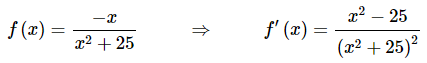Hopefully, it’s fairly clear that the critical points of the function are x=±5. We’ll leave it to you to draw a quick number line or sign chart to verify that the function will be decreasing in the range 2≤x<5 and increasing in the range x>5. Note that we just looked at the ranges of x that correspond to the ranges of n for our sequence here.
Now, because the function values are the same as the sequence values when x is an integer we can see that the sequence, which starts at n=2, has terms that increase and terms that decrease and hence the sequence is not an increasing sequence and the sequence is not a decreasing sequence. That also means that the sequence is not monotonic.
Step 2 Now let’s see what bounded information we can get.
In this case, unlike many of the previous problems in this section, we don’t have a monotonic sequence. However, we can still use the increasing/decreasing information above to help us out with the bounds.
First, we know that the sequence is decreasing in the range 2≤n<5 and increasing in the range n>5. From our Calculus I knowledge we know that this means n=5 must be a minimum of the sequence terms and hence the sequence is bounded below by −5/50=−1/10 (i.e. the n=5 sequence term).
Next let’s look for the upper bound (if it exists). For this problem let’s first take a quick look at the limit of the sequence terms. In this case the limit of the sequence terms is,
Next let’s look for the upper bound (if it exists). For this problem let’s first take a quick look at the limit of the sequence terms. In this case the limit of the sequence terms is,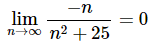Recall what this limit tells us about the behavior of our sequence terms. The limit means that as n→∞ the sequence terms must be getting closer and closer to zero.
Now, for a second, let’s look at just the portion of the sequence with n>5 and let’s further suppose that zero is not an upper bound for the sequence terms with n>5. Let’s also keep in mind that we’ve already determined that the sequence is increasing for n>5 (means that each successive term must be larger than (i.e. above) the previous one…).
So, if zero is not an upper bound (for n>5 ) then we know that somewhere there must be sequence terms with n>5 above (or larger than) zero. So, we know that terms must be getting closer and closer to zero and we’ve now assumed there are terms above zero. Therefore the only way for the terms to approach the limit of zero is for at least a few sequence terms with n>5 to decrease down towards zero (remember we’ve assumed there are terms above this!). That can’t happen however because we know that for n>5 the sequence is increasing.
Okay, what was the point of all this? Well recall that we got to this apparent contradiction to the increasing nature of the sequence for n>5 by first assuming that zero was not an upper bound for the portion of the sequence with n>5. Since making this assumption led us to something that can’t possibly be true that in turn means that zero must in fact be an upper bound for the portion of the sequence with n>5 since we’ve shown that sequence terms simply cannot go above this value!
Note that we’ve not yet actually shown that zero in an upper bound for the sequence and in fact it might not actually be an upper bound. However, what we have shown is that it is an upper bound for the vast majority of the sequence, i.e. for the portion of the sequence with n>5.
All we need to do to finish the upper bound portion of this problem off is check what the first few terms of the sequence are doing. There are several ways to do this. One is to just compute the remaining initial terms of the sequence to see if they are above or below zero. For this sequence that isn’t too bad as there are only 4 terms (n=2,3,4,5). However, if there’d been several hundred terms that wouldn’t be so easy so let’s take a look at another approach that will always be easy to do in this case because we have the increasing/decreasing information for this initial portion of the sequence.
Let’s simply note that for the first part of this sequence we’ve already shown that the sequence is decreasing. Therefore, the very first sequence term of −2/29 (i.e. the n=2 sequence term) will be the largest term for this initial bit of the sequence that is decreasing. This term is clearly less than zero and so zero will also be larger than all the remaining terms in the initial decreasing portion of the sequence and hence the sequence is bounded above by zero.
Finally, because this sequence is both bounded above and bounded below the sequence is bounded.
Before leaving this problem a couple of quick words of caution.
First, the limit of a sequence is not guaranteed to be a bound (upper or lower) for a sequence so be careful to not just always assume that the limit is an upper/lower bound for a sequence.
Second, as this problem has shown determining the bounds of a sequence can sometimes be a fairly involved process that involves quite a bit of work and lots of various pieces of knowledge about the other behavior of the sequence.

Offer running on EduRev: Apply code STAYHOME200 to get INR 200 off on our premium plan EduRev Infinity!

58 docs

,

,

,

,

,

,

,

,

,

,

,

,

,

,

,

,

,

,

,

,

,

;